# Soup

47% of pupils at the school to eat in the canteen, 1/47 of students who eat in the canteen eat lunch soup.

a) What percentage of pupils who eat in the canteen and eat soup for lunch?
b) What percentage of all pupils of school eat lunch soup?

Correct result:

a =  25 %
b =  11.75 %

#### Solution:

$a = \dfrac{1}{ 4}\cdot 100 = 25 \%$
$b = \dfrac{ 47}{100} \cdot \dfrac{1}{ 4}\cdot 100 \ \\ b = \dfrac{ 47}{100} \cdot a = 11.75 \%$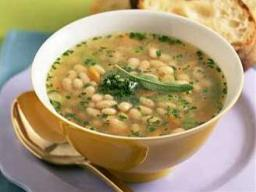We would be pleased if you find an error in the word problem, spelling mistakes, or inaccuracies and send it to us. Thank you!Tips to related online calculators
Need help calculate sum, simplify or multiply fractions? Try our fraction calculator.

## Next similar math problems:

• Soup from canteen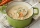For how many people is 90 liters of soup enough if we assume 3/8 liter of soup per person in the canteen?
• Raw meatIn the school cafeteria for one lunch, they prepare 490 servings of 50 g of boiled meat. Meat loses 30% of its weight by cooking. How many kilograms of raw meat must the school canteen prepare for this lunch to cook?
• Double percent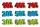What is 40% of 80% of 11800?
• Mini-surveyIn the mini-survey of our class about the popularity of individual subjects, it turned out that 11.1% of pupils like mathematics, 18.5% are enjoying languages, 30.4% of pupils like physical education, and the remaining 12 pupils have several popular subje
• Math test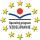In class 6.A are 26 students and the teacher had managed to give tests to 13 students. 13 testes he gave in 6.5 min. How many pupils still haven't test? How long will it take techer to give tests to 43 students?
• Report 2A School reports students to teacher ratio of 6:1. If there are 45 teachers in the School, how many students are there?
• School library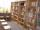The school library contains 6300 books. With this constitutes 7% of professional books for teachers 18% interest books and encyclopedias for pupils and the rest of the fairy tales. How much are which books?
• Percent calculationCalculate 51% if 99% is 79.
• LunchLunch is given to seniors from 12:15 to 12:40 during the Coronavirus pandemic. What angle will the minute hand of clock describe during this time?
• Lunch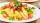Jane eats whole lunch for the 30 minutes. Which part of the lunch is eaten in 180 seconds?
• Red diplomasHe numbers of students with honors in 2013 and 2014 are in ratio 40:49. How big is the year-on-year percentage increase?
• Word problem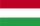348 students were on holiday in Hungary. 133 bought pizza, chips 28 pupils, 25 pupils bought soda, butter 26 pupils, 15 pupils fruits, vegetables 29 students. How much pay for all the food, if every meal cost 5.3 euros?
• StudentsAfter the fifth-grade class left 20% of students. In the seventh grade were added 2 pupils, in the eighth 1 pupil, in the ninth, the number has not changed, but it is now tenth students less than it was in the fifth grade. How many pupils are in the 9th g
• AlcoholHow many 55% alcohol we need to pour into 14 liters 75% alcohol to get p3% alcohol? How many 65% alcohol we get?
• Vinegar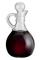We must dilute 16 liters of 8.8% aqueous vinegar to 4.1% one. How much water is necessary to add?
• Pie II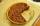Vili ate three pieces of pie. If it pieces is 1/8 how much pie did he eat?
• Summerjobs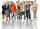The agency give summerjobs for 2352 students in 2018. The eighth was high-school students, the rest was undergraduates. How many undergraduates works via agency in 2018?